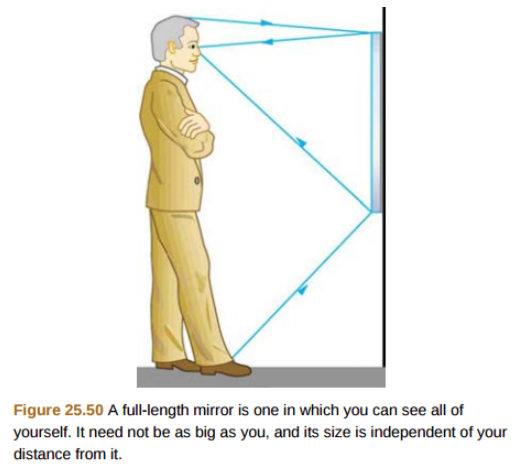# Problem: Suppose a man stands in front of a mirror as shown in Figure 25.50. His eyes are 1.65 m above the floor, and the top of his head is 0.13 m higher. Find the height above the floor of the top and bottom of the smallest mirror in which he can both the top his head and his feet. How is this distance related to the man's height?

###### FREE Expert Solution

From the law of reflection, the angle of incidence is equal to the angle of reflection.

Therefore, the top of the mirror must extend to at least half the distance between the eyes and the top of the head (let this distance be a).

Also, the bottom of the mirror must go down to at least half the distance between his eyes and the foot (let this distance be b).

The result does not depend on how far the man stands from the wall.

a = 0.13/2 = 0.065m

b = 1.65/2 = 0.825 m

99% (480 ratings)###### Problem Details

Suppose a man stands in front of a mirror as shown in Figure 25.50. His eyes are 1.65 m above the floor, and the top of his head is 0.13 m higher. Find the height above the floor of the top and bottom of the smallest mirror in which he can both the top his head and his feet. How is this distance related to the man's height?